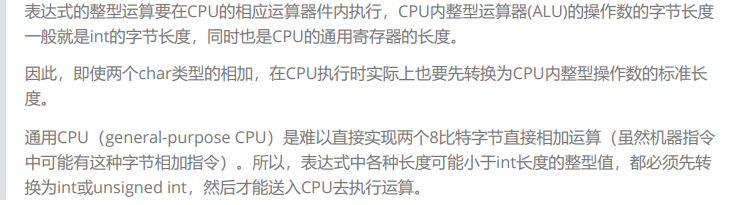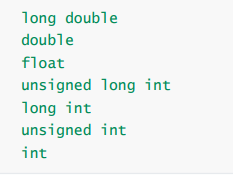﻿ 表达式求值

### 表达式求值

2021-11-22 0:37:49

1：隐式类型转换

2：算术转换

3：操作符的属性

## 1：隐式类型转换

C的整型算术运算总是至少以缺省整型类型的精度来进行的。 为了获得这个精度，表达式中的字符和短整型操作数在使用之前被转换为普通整型，这种转换称为整型提升。``````#include<stdio.h>
int main()
{
char a = 5;
// 截断
// 00000000000000000000000000000101    5的二进制 32个bit位
// 00000101  截断后存在char类型中，只有8个bit位
char b = 126;
// 00000000000000000000000001111110
// 01111110

// 00000101  - a
// 01111110  - b
// 此时整型提升
// 整形提升是按照变量的数据类型的符号位来提升的  第一位是0，补0.第一位是1，补1
// 整型提升后：      00000000000000000000000000000101  - a
//                   00000000000000000000000001111110  - b
char c = a + b;
// 此时相加          00000000000000000000000010000011  - c
// 又因为是char类型，所以取8个bit位：        10000011  - c
// 整型提升：        11111111111111111111111110000011  - c - 补码
// 内存存的是补码，打印的是原码
//                   11111111111111111111111110000010  - c - 反码
//                   10000000000000000000000001111101  - c - 原码  -125

printf("%d\n", c); // -125     整型提升
return 0;
}
``````

``````#include<stdio.h>
int main()
{
char c = 1;
printf("%u\n", sizeof(c));   //1
printf("%u\n", sizeof(+c));  //4
printf("%u\n", sizeof(-c));  //4
return 0;
}``````

## 2：算术转换`````` // char short整型提升
// int long longlong float double 算术转换
#include<stdio.h>
int main()
{
int a = 3;
float b = 5.5;
float c = a + b;  //算术转换
printf("%f\n", c);   // 8.500000
return 0;
}``````
``````// sizeof内部的表达式是不参与计算的
#include<stdio.h>
int main()
{
short s = 20;
int a = 5;
printf("%d\n", sizeof(s = a + 4));  //2
printf("%d\n", s);  //20
return 0;
}``````

## 3：操作符的属性

1. 操作符的优先级

2. 操作符的结合性

3. 是否控制求值顺序。

``````// 10.3.1操作符的优先级
#include<stdio.h>
int main()
{
int a = 10;
int b = 20;
int c = a + b * 10; //优先级
int d = a + b + 10; //相邻操作符的优先级相同的情况下，结合性说了算
return 0;
}

//一些问题表达式
//  1: a* b + c * d + e * f
//     注释：代码1在计算的时候，由于*比+的优先级高，只能保证，*的计算是比+早，但是优先级并不能决定第三个* 比第一个 + 早执行。
//  2: c + --c;
//     5 + 4 = 9
//     4 + 4 = 8
//  3:
#include<stdio.h>
int fun()
{
static int count = 1;
return ++count;
}
int main()
{